# Arcsine (asin()) in JavaScript

##### How to return the arcsine of a number in JavaScript?

The `asin()` method is nothing but the arcsine function in mathematics. Arcsine function is the inverse of sine function. This function returns the arcsine in radians of a number, and the returned value is in between -π/2 and π/2. In mathematics shortform of arcsine is 'arcsin'.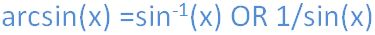In JavaScript, by using `asin()` method we can return the arcsin of a number.

NOTE: The input value of `asin()` method must be in between the -1 to 1, to return the correct arcsine of a number. If the input number is outside the range -1 to 1, the output returns 'NaN'.

### Returning the arcsine of a number in between the range 1 to -1

```<p>Click the below button to return arcsine value</p>
<input type="button" onclick="myNumber()" value="Click">
<p id="myId"></p>

<script>
function myNumber() {
document.getElementById("myId").innerHTML = Math.asin(-0.3);
}
</script>
```

In the above code snippet we have given `Id` as "`myId`"to the second `<p>` element in the HTML code. There is a function myNumber() in the`<script>`block which is connected to the onclick of the HTML button. We need to return the arcsine of a number, for that we are using `asin()` method. Onclick of the button "Click" in the HTML code fires the function myNumber() in the `<script>` block at the same time `Math.asin()` method returns the arcsine value of a given input number as output.

OUTPUT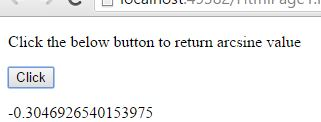### Returning the arcsine of different values

```<p>Click the below button to return arcsine value</p>
<input type="button" onclick="myFunction()" value="Click">
<p id="myId"></p>

<script>
function myFunction() {
var a = Math.asin(0.3);
var b = Math.asin(-0.3);
var c = Math.asin(-1.3);
var d = Math.asin(1.3);

var r = "The arcsine value of 0.3 is :" + a
+"<br>" + "The arcsine value of -0.3 is :" + b
+ "<br>" + "The arcsine value of -1.3 is :" + c
+ "<br>" +"The arcsine value of 0.3 is :" + d;
document.getElementById("myId").innerHTML = r;
}
</script>

<p><strong>Note:</strong>If the input value of arcsine method is not in range between -1 to 1, the output shows NaN.</p>
```

Arcsine of different values

Onclick of the button "Click" in the HTML code fires the function myFunction() in the `<script>` block at the same time `Math.asin()` method returns the arcsine values of a given input numbers as output.

OUTPUT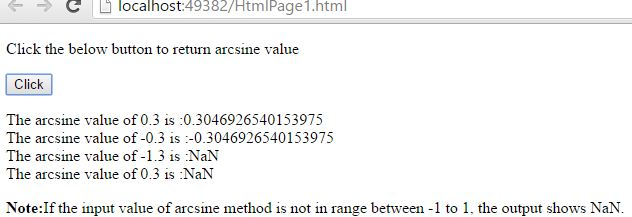### Returning the arcsine value of the number enetered by the user

```<input type="button" onclick="myNumber()" value="Click to Enter Vlue">

<script>
function myNumber() {
val = prompt("Enter the value in between -1 to +1 to know the exact Arcsine value:", "");
alert('Arcsine value of entered number is::' + Math.asin(val));
}
</script>
```

Returning the arcsine value of the number enetered by the user

In the above code snippet there is a function myNumber() in the `<script>` block which is connected to the onclick of the HTML button. We need to return the arcsine value of a number which is entered by the user, for that we are using `Math.asin(val)` method in the alert box. Onclick of the button in the HTML code fires the function in the `<script>` block at same time user asked to enter the value in the input box, after entering the value and then clicking on 'OK' button gives the arcsine value of a entered number as output in the alert box.

OUTPUT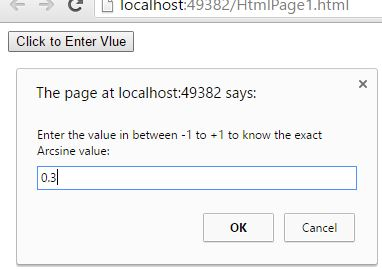In the above output after clicking the 'Click to Enter Value' button, the user asked to enter the input value. The user entered the input value '0.3' in the above output, after entering the input value click on 'OK' button to know the arcsine value of entered number.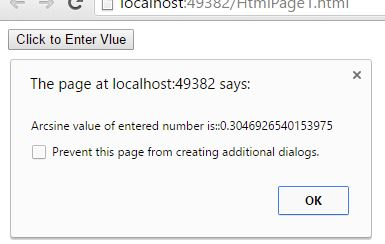Views: 4267 | Post Order: 97